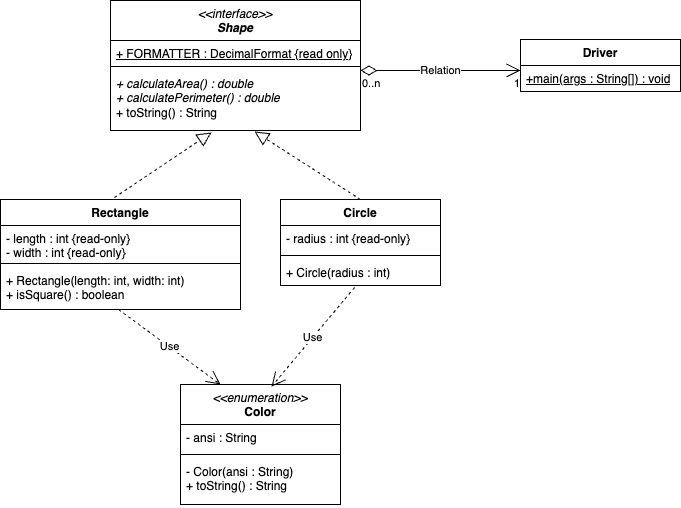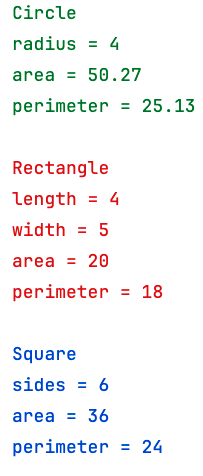# Shapes

In Java, an `Interface` is used to define the public methods that are shared by a given set of `Object`s.They are used to standardize the communication between classes, requiring all abstract methods declared in the interface to be fully implemented in the implementing classes#### The Shape Interface

The `Shape` interface defines three methods that must be implemented in all shapes that implement this interface: `calculateArea(),` `calculatePerimeter()`, and `toString()`. In addition, there is a constant `DecimalFormat` object that will be shared among all instances of the implementing classes.

#### Implementing Classes

There are two implementing classes, `Circle` and `Rectangle`, that will fully implement all three of those defined methods. Note that because these classes implement the `Shape` interface, they may be referenced either as their class or as a `Shape`. Use your `Color` enumeration from the previous homework to format the output.

#### Driver class

The driver class will contain only the `main()` method and should do the following:

• Instantiate three different `Shape`s
• A `Circle` will a radius of 4
• A `Rectangle` with length of 4 and a width of 5
• A Square(`Rectangle`) with sides of 6
• Store all of these `Shape`s in an `ArrayList`
• Using a foreach loop, print each of these `Shape`s to the console
• The output should exactly match the sample output below

#### Sample Output